# Algebra II : Hyperbolic Functions

## Example Questions

2 Next →

### Example Question #1021 : Algebra Ii

Which of the following shapes does the graph of the equation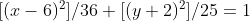take?

Hyperbola

Parabola

Ellipse

Circle

Ellipse

Explanation:

The equation for a horizontal hyperbola is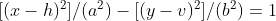. The equation for a vertical hyperbola is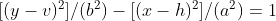. Both include x- and y-terms combined using subtraction. The equation for an ellipse is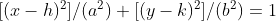. Because the given equation connects the x- and y-terms using addition rather than subtraction, it represents an ellipse rather than a hyperbola. If the equation took the form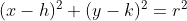, it would represent a circle. If the equation took the form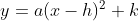, it would represent a parabola.

### Example Question #1022 : Algebra Ii

Which of the following shapes does the graph of the equation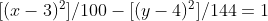take?

Circle

Hyperbola

Ellipse

Parabola

Hyperbola

Explanation:

The equation for a horizontal hyperbola is. The equation for a vertical hyperbola is. Both include x- and y-terms combined using subtraction. If the equation took the form(using addition rather than subtraction to combine the x- and y-terms), it would represent an ellipse. If the equation took the form, it would represent a circle. If the equation took the form, it would represent a parabola.

### Example Question #11 : Hyperbolic Functions

What are the coordinates of the center of the hyperbolic inequality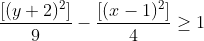?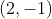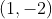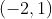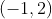Explanation:

The equation for a horizontal hyperbola is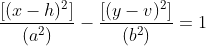. The equation for a vertical hyperbola is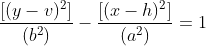. In both, (h, v) is the center of the hyperbola. Hyperbolic inequalities use an inequality sign rather than an equals sign, but otherwise have the same form as hyperbolic equations. Because the two terms are combined using subtraction and the y-term appears first, this inequality represents a vertical hyperbola. To derive the center of a hyperbola from its equation or inequality, flip the sign of the constants that appear after the x and y in the equation or inequality. The constant following x is -1, so the x-coordinate of the center is 1. The constant following y is 2, so the y-coordinate of the center is -2.

The graph of the hyperbolic inequalityappears as follows: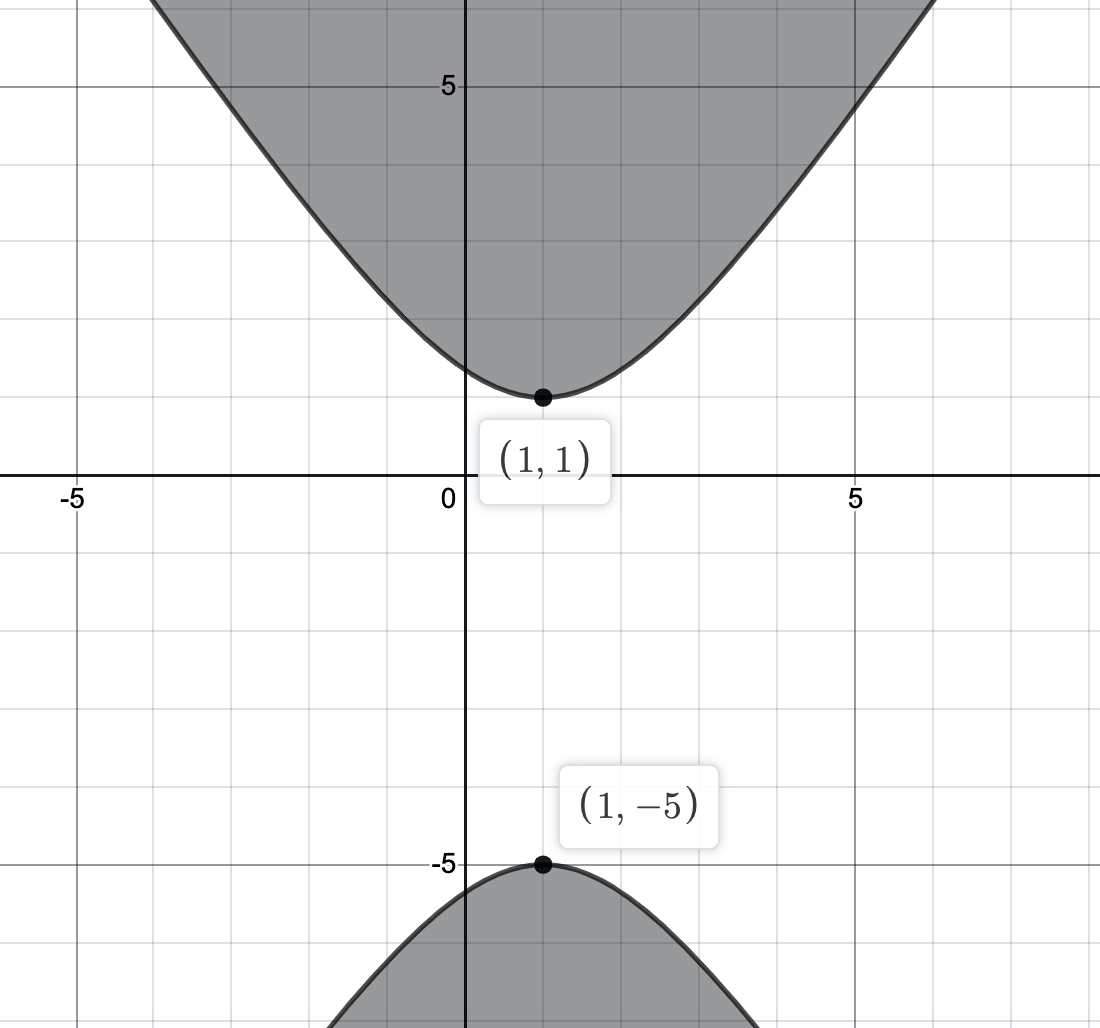With vertices of (1, 1) and (1, -5), you can see that the midpoint between them is (1, -2).

### Example Question #12 : Hyperbolic Functions

What are the coordinates of the center of the hyperbolic inequality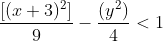?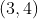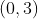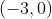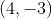Explanation:

The equation for a horizontal hyperbola is. The equation for a vertical hyperbola is. In both, (h, v) is the center of the hyperbola. Hyperbolic inequalities use an inequality sign rather than an equals sign, but otherwise have the same form as hyperbolic equations. Because the two terms are combined using subtraction and the x-term appears first, this inequality represents a horizontal hyperbola. To derive the center of a hyperbola from its equation or inequality, flip the sign of the constants that appear after the x and y in the equation or inequality. The constant following x is 3, so the x-coordinate of the center is -3. No constant follows y, so the y-coordinate of the center is 0.

The graph of the hyperbolic inequalityappears as follows: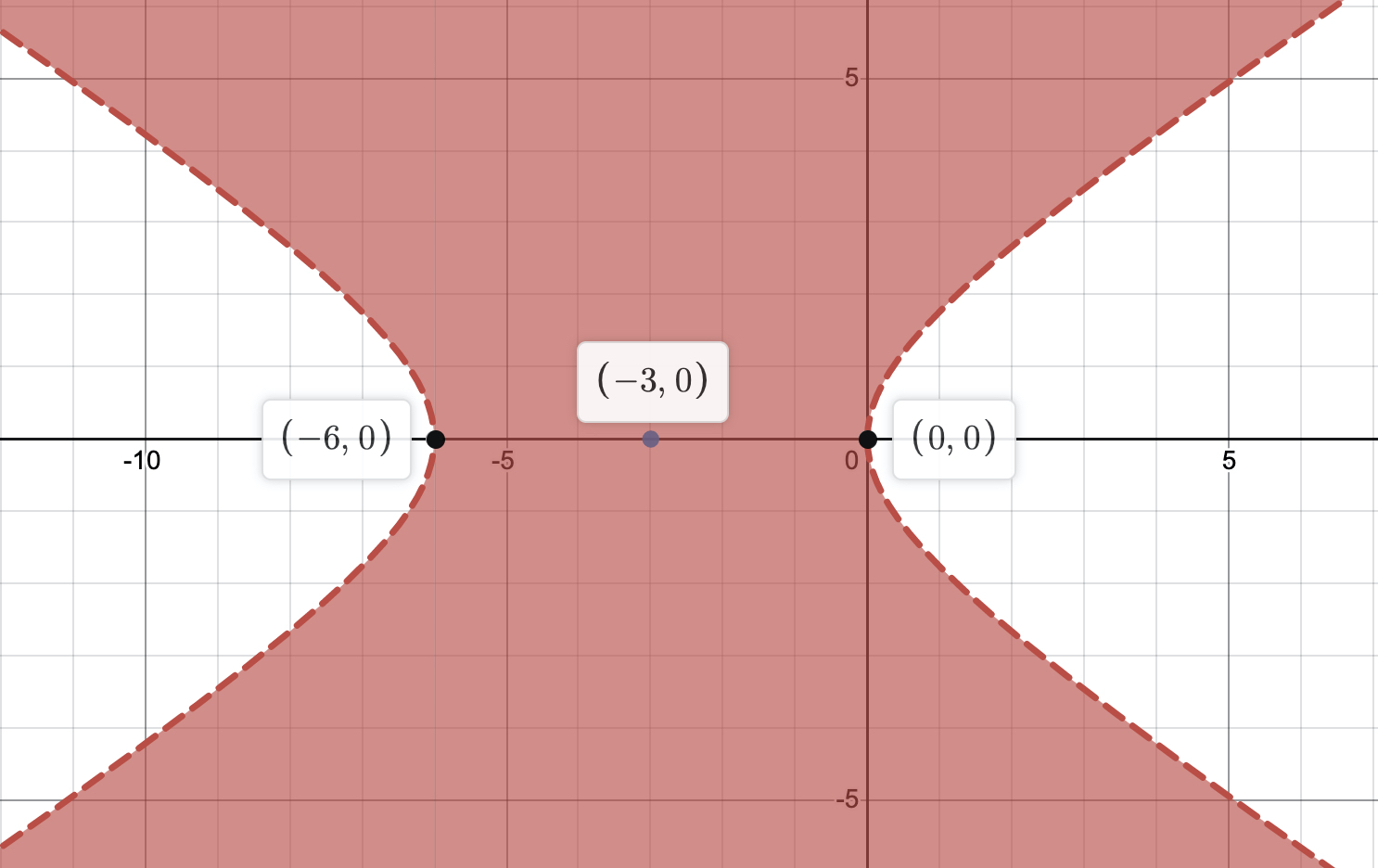As you can see, the midpoint between the two vertices of (-6, 0) and (0, 0) is (-3, 0).

### Example Question #13 : Hyperbolic Functions

Which equation does this graph represent?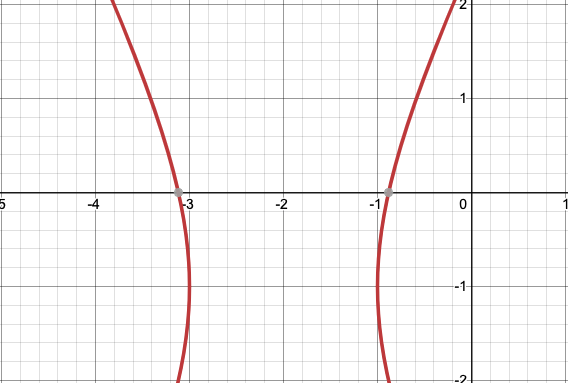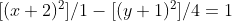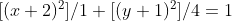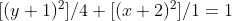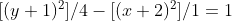Explanation:

The equation for a horizontal hyperbola is. The equation for a vertical hyperbola is. In both, (h, v) is the center of the hyperbola. The graph shows a horizontal hyperbola, so in its corresponding equation the x-term must appear first. The center lies at (-2, -1), so x must be followed by the constant 2, and y must be followed by the constant 1. The graph shows a hyperbola rather than an ellipse, so the x- and y-terms must be combined using subtraction rather than addition.

### Example Question #11 : Hyperbolic Functions

In which direction does the graph of the hyperbolaopen?

vertical

horizontal

horizontal

Explanation:

The equation for a horizontal hyperbola is. The equation for a vertical hyperbola is. The x-term appears first, so the given equation represents a horizontal hyperbola.

2 Next →

### All Algebra II Resources